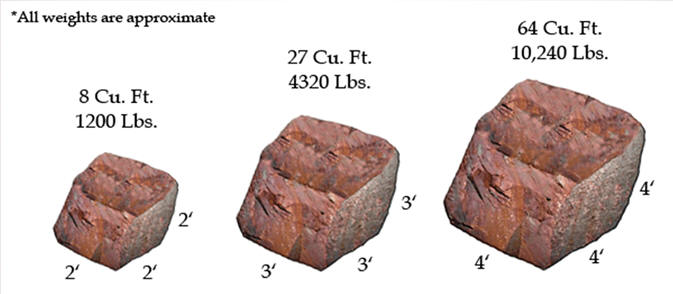# Boulder Weight Estimation Guide

The average weight of a sandstone boulder is approximately 150 pounds per cubic foot. Limestone boulders and granite boulders in most cases weigh more. They average about 175 pounds per cubic foot. To estimate the quantity, you need to convert the area into cubic feet by multiplying length x width x height. Once you have this number, multiply it by the boulder's weight per cubic foot, then divide by 2000 to convert this number into tons.